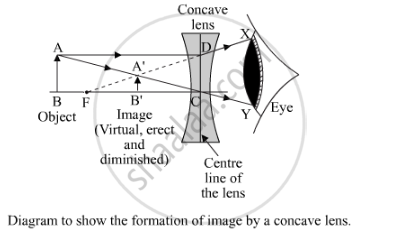Share

# A Small Object is Placed 150 Mm Away from a Diverging Lens of Focal Length 100 Mm. (I) Copy the Figure Below and Draw Rays to Show How an Image is Formed by the Lens. Figure (Ii) Calculate the Distance of the Image from the Lens by Using the Lens Formula. - CBSE Class 10 - Science

ConceptReflection of Light Spherical Mirrors

#### Question

A small object is placed 150 mm away from a diverging lens of focal length 100 mm.
(i) Copy the figure below and draw rays to show how an image is formed by the lens.
Figure
(ii) Calculate the distance of the image from the lens by using the lens formula.

#### Solution

The image formed by the lens is virtual, erect and diminished in size.(a)(ii) Object distance (u) = -150 mm = -15 cm (sign convention)
Focal length (f) = -100 mm = -10 cm (sign convention)
Image distance (v) = ?

Applying the lens formula, we have:

1/v-1/u=1/f

1/v-1/-15=1/-10

1/v+1/15=-1/10

1/v=1/10-1/15

1/v=(-3-2)/30

1/v=(-5)/30

v=-6 cm

Is there an error in this question or solution?

#### Video TutorialsVIEW ALL 

Solution A Small Object is Placed 150 Mm Away from a Diverging Lens of Focal Length 100 Mm. (I) Copy the Figure Below and Draw Rays to Show How an Image is Formed by the Lens. Figure (Ii) Calculate the Distance of the Image from the Lens by Using the Lens Formula. Concept: Reflection of Light - Spherical Mirrors.
S